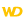Home |Sign in | EnglishHelpDrawRectangleGradientPresentationExampleGradient parametersDrawing in Browser codeRelated examplesSee alsoAlphaBlendBackgroundChangeModeDrawChordDrawLineDrawPointDrawPolygonDrawPolylineDrawRectangleDrawRectangleGradientDrawRoundedRectangleDrawSliceDrawTextDrawTextRTFEndDrawingFillFontHorizontalSymmetryInvertColorPenPixelColorPixelOpacityResizeRotationStartDrawingVerticalSymmetryNew WINDEV, WEBDEV 25 feature!
• Drawing in Browser code
WINDEVWEBDEVWINDEV MobileOthersDraws a rectangle in an picLayer variable.Versions 15 and laterThis function is now available for the Java applications.This function is now available for WEBDEV sites in Linux.New in version 15This function is now available for the Java applications.This function is now available for WEBDEV sites in Linux.This function is now available for the Java applications.This function is now available for WEBDEV sites in Linux.Versions 16 and laterThis function is now available for WINDEV applications in Linux.New in version 16This function is now available for WINDEV applications in Linux.This function is now available for WINDEV applications in Linux.
Example
MyWDPicImage is WDPic = "Test.gif"
MyLayer is picLayer

// Draw a rectangle whose background changes from red to blue
// via yellow
MyLayer.DrawRectangleGradient(10, 20, 100, 150, LightRed, LightBlue, 0, LightYellow, 30)
IMG_MyDrawing = MyWDPicImage
Syntax

Drawing a rectangle by specifying the points of the rectangle and the gradient

<picLayer image>.DrawRectangleGradient(<X1> , <Y1> , <X2> , <Y2> , <Start color> , <End color> [, <Angle> [, <Color 3> [, <Distance color 3> [, <Color 4> [, <Distance color 4>]]]]])
<picLayer image>: picLayer variable
Name of the picLayer variable to use.
<X1>: Integer
X coordinate (in pixels) of top left corner of rectangle.
<Y1>: Integer
Y coordinate (in pixels) of top left corner of rectangle.
<X2>: Integer
X coordinate (in pixels) of bottom right corner of rectangle.
<Y2>: Integer
Y coordinate (in pixels) of bottom right corner of rectangle.
<Start color>: Integer or constant
Start color of gradient. This color can correspond to:
<End color>: Integer or constant
End color of gradient. This color can correspond to:
<Angle>: Optional integer
Angle that will be used for the selection of colors in the gradient. The angle value is included between 0 (horizontal angle, default value) and 360°.
<Color 3>: Optional constant or integer
Color that will be used in the gradient. This color can correspond to:
<Distance color 3>: Optional integer
Percentage separating the start color from color 3.
<Color 4>: Optional constant or integer
Color that will be used in the gradient. This color can correspond to:
<Distance color 4>: Optional integer
Percentage separating the start color from color 4.Drawing a rectangle by specifying the points of the rectangle and by using the Background type

<picLayer image>: picLayer variable
Name of the picLayer variable to use.
<X1>: Integer
X coordinate (in pixels) of top left corner of rectangle.
<Y1>: Integer
Y coordinate (in pixels) of top left corner of rectangle.
<X2>: Integer
X coordinate (in pixels) of bottom right corner of rectangle.
<Y2>: Integer
Y coordinate (in pixels) of bottom right corner of rectangle.
Name of the Background variable the with the gradient characteristics.Drawing a rectangle by using the Rectangle type and by specifying the gradient

<picLayer image>.DrawRectangleGradient(<Rectangle> , <Start color> , <End color> [, <Angle> [, <Color 3> [, <Distance color 3> [, <Color 4> [, <Distance color 4>]]]]])
<picLayer image>: picLayer variable
Name of the picLayer variable to use.
<Rectangle>: Rectangle variable
Name of the Rectangle variable that defines the coordinates of the rectangle to draw.
<Start color>: Integer or constant
Start color of gradient. This color can correspond to:
<End color>: Integer or constant
End color of gradient. This color can correspond to:
<Angle>: Optional integer
Angle that will be used for the selection of colors in the gradient. The angle value is included between 0 (horizontal angle, default value) and 360°.
<Color 3>: Optional constant or integer
Color that will be used in the gradient. This color can correspond to:
<Distance color 3>: Optional integer
Percentage separating the start color from color 3.
<Color 4>: Optional constant or integer
Color that will be used in the gradient. This color can correspond to:
<Distance color 4>: Optional integer
Percentage separating the start color from color 4.Drawing a rectangle by using the Rectangle type and the Background type

<picLayer image>: picLayer variable
Name of the picLayer variable to use.
<Rectangle>: Rectangle variable
Name of the Rectangle variable that defines the coordinates of the rectangle to draw.
Name of the Background variable the with the gradient characteristics.
Remarks

The color parameters are used as follows:The angle is used to define the orientation of the gradient.Gradients with 3 or 4 colors are only available for 0 or 90 degree angles.
Remark: The gradient colors are not available for some systems (Windows 98 or Me for example) or for TSE 256 colors. In this case, a rectangle whose color corresponds to <Start color> is displayed.
Related Examples:Unit examples (WINDEV): The drawing functions [ + ] Using the main drawing functions of WINDEV to:- Initialize an Image control for drawing- Draw simple shapes- Write a text into a drawing- Change the color in a drawing
Business / GUI classification : Neutral code
Component : wd250pnt.dll
Minimum version required
• Version 25